••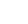##### SkillUrSelf
•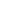##### VideoCourse
•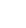##### OnlineTest Series
•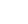##### StudyMaterial
•##### TestUrSelfBlog
•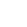### How to Study Engineering Mathematics for GATE 2020 Exam Effectively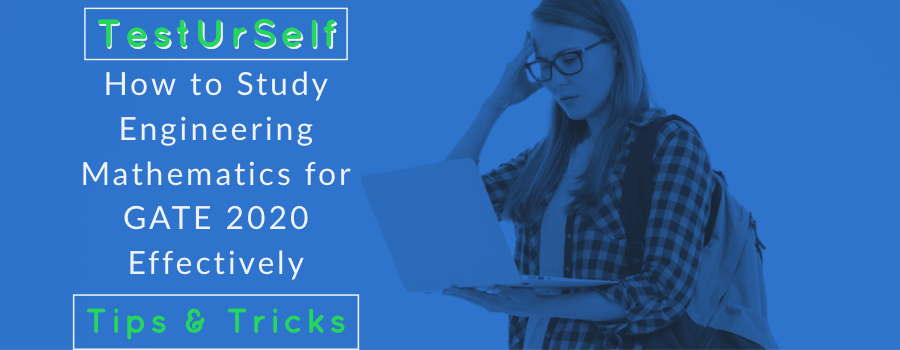This Topic is very important for the GATE Exam. It covers around 13-15 Marks out of 100. You can easily score minimum 10 marks in this section if you can manage to prepare well.

The key to prepare for this subject is to make short notes which have only formulae/graphs/concepts of at most importance, especially note down the area which you find difficult to remember them and keep them handy with you or have short notes so that you can look up to a concept whenever you need it.

Which Book/Study Materials is the good for the GATE Exam?

You can follow Higher Engineering Mathematics by B. S. Grewal. If you are in 2nd or 3rd year then we will be recommended you that to follow this book otherwise go with TestUrSelf Digital Study Materials for Engineering Mathematics. Because it will included 100+ Quality Solved examples and also you will get 10+ Online Assignment as mentioned below:

Details of the TestUrSelf Study Materials for Engineering Mathematics:

 Lecture Name Number of Online Assignment TeachUrSelf Linear Algebra 2 TeachUrSelf Calculus 1 TeachUrSelf Vector Calculus 2 TeachUrSelf Differential Equations 2 TeachUrSelf Complex Variable 1 TeachUrSelf Probability & Statistics 2 TeachUrSelf Numerical Methods 2

NoteThe Study Materials for Engineering Mathematics is Live Now.

Take a look at the topic-wise strategy to prepare for engineering mathematics in GATE 2020 Exam.

Linear Algebra (For all Streams): very Important

While preparing for Linear Algebra, the focus must be on Eigenvalue and Eigen Vectors Problems and also the Matrix Algebra as almost every-time there will be a question from either of these two topics. One must also remember the properties of eigenvalues like the relation between eigenvalues of two matrices which are inverse of each other and other similar properties.

Calculus (For all Streams):

This topic has been divided into 3 categories:

• Differential Calculus: It involves basic concepts of Limits, Derivative and application of derivatives such as Increasing, Decreasing function and Maxima and Minima problems.
• Integral Calculus: It involves indefinite and definite integral and the properties of definite integrals. Also, you need to be very well versed with the concepts of multiple integrals like Double and Triple Integral and also Line, Surface and Volume Integrals.
• Vector Calculus (very important topic): It involves Gradient, Divergence and Curl and Vector Integral Theorems (Green’s, Gauss’s and Stokes Theorem) and there are lot many properties of each concept and you must remember all those to solve the problems faster like divergence of curl is always zero, believe me, that these properties do come in handy in the exam.

Differential Equations (For all Streams):

• Finding the solution of differential equations are extremely important. Focusing on higher order differential equations is a must along with first order equations. Bernoulli’s Equation and Euler Differential Equation should also be paid attention to.
• Also, in the latest years questions based on the existence of solution and uniqueness theorem have been seen so they also need to be prepared.

Probability and Statistics (For all Streams): Very Important

• The general problems involving Probability can be prepped up by practice and there is no need to study much about them but you can always remember Baye’s Theorem, more focus should be on Random Variables like Poisson’s Distribution and other distributions. Statistics is also very important and you must remember to mean, median and mode concepts as well as coefficient of co-relation, so do make short notes for each of these concepts.

Complex Variables (Excluding MT & CS):

• It is the smallest topic among all topics from Engineering Mathematics and the only important concepts are Cauchy-Riemann Equations for Analytic Functions and Residue Method of Integration and rest of the topics are not necessary for GATE.

Numerical Methods (Excluding CS): very Important

• Two things are important in this section one is the equation solving by methods like Newton-Raphson and Bisection Method and other is the Numerical Integration technique by methods like Trapezoidal Rule and Simpson’s rule so do mug up the formulas for each of these methods and make good use of Calculator like you can form equations in the form of answer of the previous step so that recursion becomes faster and more accurate.

Transform Theory (For EE and ME ONLY):

• Laplace and inverse Laplace transforms are the topics to be practiced well.

You can see the given below Pi chart for the distribution of questions which are asked in every year.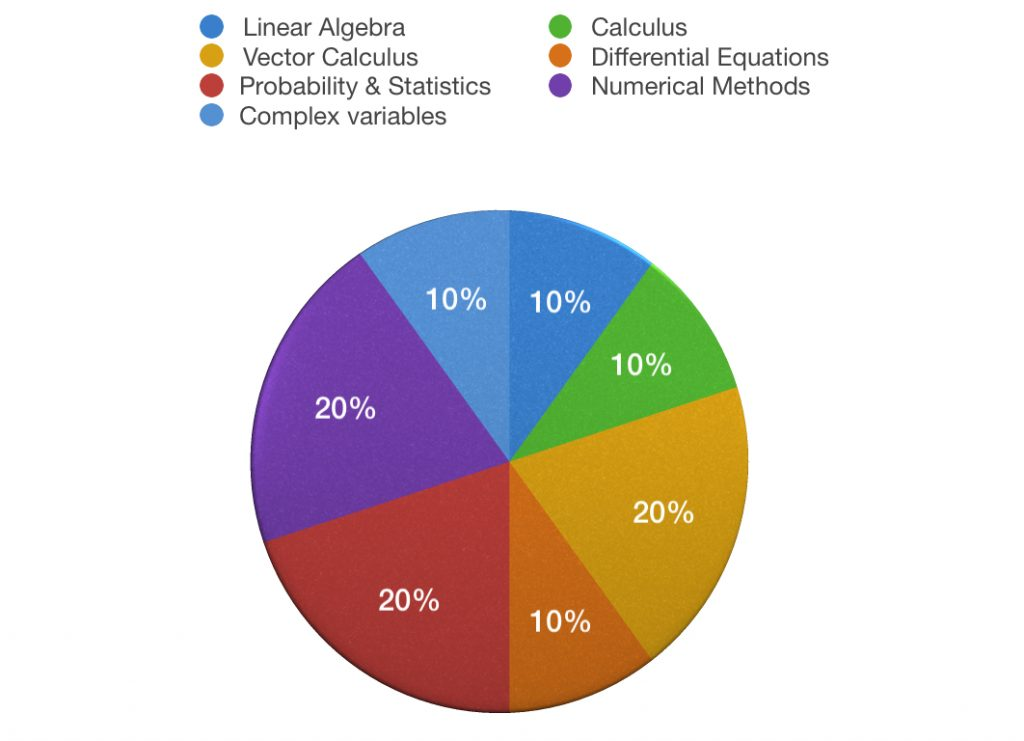Some Important tips:

• Do not try to memorize maths by mugging up the entire textbooks/standard books. You’ll only be wasting time. Remember the formulae and understand the techniques used to solve the questions.
• Practice previous year numerical after you complete each topic and use virtual calculator while solving them.
• Revise regularly. Revise the formulae every day. Solve a few questions from the topics.
• You should take mock tests. You should join a good Online Test Series which will help you in practicing the questions in a simulated environment.
• Make a Short Notes. It is essential for last moment revision.

Try TestUrSelf & compete with your fellow aspirants and ace RANK below 100.

All the best for Ur preparation!

-TestUrSelf Team

##### TestUrSelf

We are very proud to say that TestUrSelf is the ONLY test series for GATE which provides not only GATE relevant questions but also very advanced performance analysis. You can search the internet about the reviews.

Prepare for GATE in one of the most effective and result-oriented ways with TestUrSelf free and paid courses.